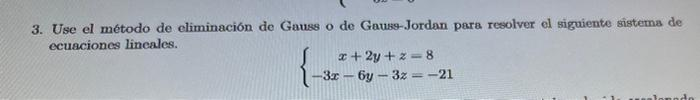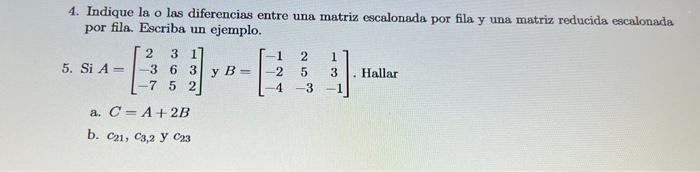# Question 3. Use el método de eliminación de Gauss o de Gauss-Jordan para resolver el siguiente sistema de ecuaciones lineales. $\left\{\begin{array}{c} x+2 y+z=8 \\ -3 x-6 y-3 z=-21 \end{array}\right.$ 4. Indique la o las diferencias entre una matriz escalonada por fila y una matriz reducida escalonada por fila. Escriba un ejemplo. 5. Si $$3. Use el método de eliminación de Gauss o de Gauss-Jordan para resolver el siguiente sistema de ecuaciones lineales. $\left\{\begin{array}{c} x+2 y+z=8 \\ -3 x-6 y-3 z=-21 \end{array}\right.$ 4. Indique la o las diferencias entre una matriz escalonada por fila y una matriz reducida escalonada por fila. Escriba un ejemplo. 5. Si \( A=\left[\begin{array}{ccc}2 & 3 & 1 \\ -3 & 6 & 3 \\ -7 & 5 & 2\end{array}\right]$$ y $$B=\left[\begin{array}{ccc}-1 & 2 & 1 \\ -2 & 5 & 3 \\ -4 & -3 & -1\end{array}\right]$$. Hallar a. $$C=A+2 B$$ b. $$c_{21}, c_{3,2}$$ y $$c_{23}$$Transcribed Image Text: 3. Use el método de eliminación de Gauss o de Gauss-Jordan para resolver el siguiente sistema de ecuaciones lineales. $\left\{\begin{array}{c} x+2 y+z=8 \\ -3 x-6 y-3 z=-21 \end{array}\right.$ 4. Indique la o las diferencias entre una matriz escalonada por fila y una matriz reducida escalonada por fila. Escriba un ejemplo. 5. Si $$A=\left[\begin{array}{ccc}2 & 3 & 1 \\ -3 & 6 & 3 \\ -7 & 5 & 2\end{array}\right]$$ y $$B=\left[\begin{array}{ccc}-1 & 2 & 1 \\ -2 & 5 & 3 \\ -4 & -3 & -1\end{array}\right]$$. Hallar a. $$C=A+2 B$$ b. $$c_{21}, c_{3,2}$$ y $$c_{23}$$
Transcribed Image Text: 3. Use el método de eliminación de Gauss o de Gauss-Jordan para resolver el siguiente sistema de ecuaciones lineales. $\left\{\begin{array}{c} x+2 y+z=8 \\ -3 x-6 y-3 z=-21 \end{array}\right.$ 4. Indique la o las diferencias entre una matriz escalonada por fila y una matriz reducida escalonada por fila. Escriba un ejemplo. 5. Si $$A=\left[\begin{array}{ccc}2 & 3 & 1 \\ -3 & 6 & 3 \\ -7 & 5 & 2\end{array}\right]$$ y $$B=\left[\begin{array}{ccc}-1 & 2 & 1 \\ -2 & 5 & 3 \\ -4 & -3 & -1\end{array}\right]$$. Hallar a. $$C=A+2 B$$ b. $$c_{21}, c_{3,2}$$ y $$c_{23}$$